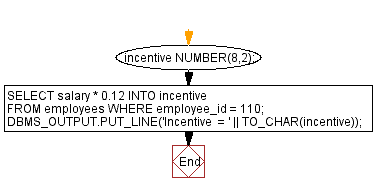﻿ PL/SQL Fundamentals Exercises: PL/SQL block to calculate incentive assigning values to variable - w3resource# PL/SQL Fundamentals Exercises: Calculate the incentive of an employee whose ID is 110

## PL/SQL Fundamentals: Exercise-1 with Solution

Write a PL/SQL block to calculate the incentive of an employee whose ID is 110.

The following PL/SQL block shows a veriable incentive have been declared and incentive have assigned into this variable.

Sample Solution:

PL/SQL Code:

``````DECLARE
incentive   NUMBER(8,2);
BEGIN
SELECT salary * 0.12 INTO incentive
FROM employees
WHERE employee_id = 110;
DBMS_OUTPUT.PUT_LINE('Incentive  = ' || TO_CHAR(incentive));
END;
/
``````

Sample Output:

```Incentive  = 984

Statement processed.

0.00 seconds
```

Flowchart:Improve this sample solution and post your code through Disqus

What is the difficulty level of this exercise?

﻿